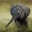Related Tags

c++

# Split a linked list into two sublists with alternating elementsChayshta Singh

### Overview

The following article will go through how to split a linked list into two sub-lists with each containing alternate elements from the original list.

### Algorithm

Append the current element to one of the two sub-lists while traversing the original list, list 1. We will use a boolean variable to determine whether the entry is added to list 2 or list 3. This variable value toggles between true and false with each iteration.

The following are the two points to be considered in the solution:

1. Function signature: Use reference to a pointer.
2. Fix the tail of list 2 and list 3 to point to nullptr.

### Code

Node.h

#include<iostream>
#include "Node.h"
using namespace std;

// head is a pointer to original list
// head1 is a reference to a pointer to sublist 1
// head2 is a reference to a pointer to sublist 2
Node *current1 = nullptr, *current2 = nullptr;
bool alternate = true;
while(current){
if(alternate){
// add element in list1 for alternate = true
if(current1){
current1->next = current;
current1 = current1->next;
}
else
} else {
// add element in list2 for alternate = false
if(current2){
current2->next = current;
current2 = current2->next;
}
else
}
current = current->next;
alternate = !alternate;
}
//fix the tail pointers of list1 and list2
if(current1)
current1->next = nullptr;
if(current2)
current2->next = nullptr;
}

//list1 will contain these values.
int arr[] = {1,2,3,4,5,6,7,8,9};
int size = 9;


Code

### Time complexity analysis

The algorithm's time complexity is O(N), where N is the length of the original linked list.

### Explanation

• Lines 16–22: This section works only for true values of the alternate variable. Data of the current pointer is appended to current1. The head1 keyword is initialized if it’s the first element in the list.
• Lines 24–29: This section work only for false values of the alternate variable. Data of the current pointer is appended to list2. Then, the head2 keyword is initialized if it’s the first element in the list.
• Line 36: If current1 is not the first entry in the list1, we will set its next value to nullptr after appending.
• Line 38: If current2 is not the first entry in the list2, we will set its next value to nullptr after appending.

RELATED TAGS

c++

CONTRIBUTORChayshta Singh
RELATED COURSES

View all Courses

Keep Exploring

Learn in-demand tech skills in half the time